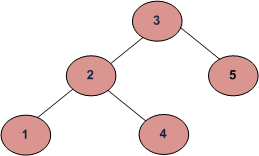Related Articles

# Print Levels of all nodes in a Binary Tree

• Difficulty Level : Easy
• Last Updated : 30 Jun, 2021

Given a Binary Tree and a key, write a function that prints levels of all keys in given binary tree.
For example, consider the following tree. If the input key is 3, then your function should return 1. If the input key is 4, then your function should return 3. And for key which is not present in key, then your function should return 0.```Input:
3
/ \
2   5
/ \
1   4

output:
Level of 1 is 3
Level of 2 is 2
Level of 3 is 1
Level of 4 is 3
Level of 5 is 2```

We have discussed an recursive solution in below post.
Get Level of a node in a Binary Tree
In this post, an iterative solution based on Level order traversal is discussed. We store level of every node in queue together with the node while doing the traversal.

## C++

 `// An iterative C++ program to print levels``// of all nodes``#include ``using` `namespace` `std;` `/* A tree node structure */``struct` `Node {``    ``int` `data;``    ``struct` `Node* left;``    ``struct` `Node* right;``};` `void` `printLevel(``struct` `Node* root)``{``    ``if` `(!root)``        ``return``;` `    ``// queue to hold tree node with level``    ``queue > q;` `    ``q.push({root, 1}); ``// let root node be at level 1` `    ``pair<``struct` `Node*, ``int``> p;` `    ``// Do level Order Traversal of tree``    ``while` `(!q.empty()) {``        ``p = q.front();``        ``q.pop();` `        ``cout << ``"Level of "` `<< p.first->data``             ``<< ``" is "` `<< p.second << ``"\n"``;` `        ``if` `(p.first->left)``            ``q.push({ p.first->left, p.second + 1 });``        ``if` `(p.first->right)``            ``q.push({ p.first->right, p.second + 1 });``    ``}``}` `/* Utility function to create a new Binary Tree node */``struct` `Node* newNode(``int` `data)``{``    ``struct` `Node* temp = ``new` `struct` `Node;``    ``temp->data = data;``    ``temp->left = temp->right = NULL;``    ``return` `temp;``}` `/* Driver function to test above functions */``int` `main()``{``    ``struct` `Node* root = NULL;` `    ``/* Constructing tree given in the above figure */``    ``root = newNode(3);``    ``root->left = newNode(2);``    ``root->right = newNode(5);``    ``root->left->left = newNode(1);``    ``root->left->right = newNode(4);` `    ``printLevel(root);``    ``return` `0;``}`

## Java

 `// Java program to print``// levels of all nodes``import` `java.util.LinkedList;``import` `java.util.Queue;``public` `class` `Print_Level_Btree {``    ` `    ``/* A tree node structure */``    ``static` `class` `Node {``        ``int` `data;``        ``Node left;``        ``Node right;``        ``Node(``int` `data){``            ``this``.data = data;``            ``left = ``null``;``            ``right = ``null``;``        ``}``    ``}``    ` `    ``// User defined class Pair to hold``    ``// the node and its level``    ``static` `class` `Pair{``        ``Node n;``        ``int` `i;``        ``Pair(Node n, ``int` `i){``            ``this``.n = n;``            ``this``.i = i;``        ``}``        ` `    ``}``    ` `    ``// function to print the nodes and``    ``// its corresponding level``    ``static` `void` `printLevel(Node root)``    ``{``        ``if` `(root == ``null``)``            ``return``;``     ` `        ``// queue to hold tree node with level``        ``Queue q = ``new` `LinkedList();``     ` `        ``// let root node be at level 1``        ``q.add(``new` `Pair(root, ``1``));``     ` `        ``Pair p;``     ` `        ``// Do level Order Traversal of tree``        ``while` `(!q.isEmpty()) {``            ``p = q.peek();``            ``q.remove();``     ` `            ``System.out.println(``"Level of "` `+ p.n.data +``                    ``" is "` `+ p.i);``            ``if` `(p.n.left != ``null``)``                ``q.add(``new` `Pair(p.n.left, p.i + ``1``));``            ``if` `(p.n.right != ``null``)``                ``q.add(``new` `Pair(p.n.right, p.i + ``1``));``        ``}``    ``}``    ` `    ``/* Driver function to test above``        ``functions */``    ``public` `static` `void` `main(String args[])``    ``{``        ``Node root = ``null``;``     ` `        ``/* Constructing tree given in the``              ``above figure */``        ``root = ``new` `Node(``3``);``        ``root.left = ``new` `Node(``2``);``        ``root.right = ``new` `Node(``5``);``        ``root.left.left = ``new` `Node(``1``);``        ``root.left.right = ``new` `Node(``4``);``     ` `        ``printLevel(root);``    ``}``}``// This code is contributed by Sumit Ghosh`

## Python3

 `# Python3 program to print levels``# of all nodes` `# Helper function that allocates a new``# node with the given data and None``# left and right poers.                                    ``class` `newNode:` `    ``# Construct to create a new node``    ``def` `__init__(``self``, key):``        ``self``.data ``=` `key``        ``self``.left ``=` `None``        ``self``.right ``=` `None` `def` `prLevel( root):` `    ``if` `(``not` `root):``        ``return` `    ``# queue to hold tree node with level``    ``q ``=` `[]` `    ``# let root node be at level 1``    ``q.append([root, ``1``])` `    ``p ``=` `[]` `    ``# Do level Order Traversal of tree``    ``while` `(``len``(q)):``        ``p ``=` `q[``0``]``        ``q.pop(``0``)``        ``print``(``"Level of"``, p[``0``].data, ``"is"``, p[``1``])``        ``if` `(p[``0``].left):``            ``q.append([p[``0``].left, p[``1``] ``+` `1``])``        ``if` `(p[``0``].right):``            ``q.append([p[``0``].right, p[``1``] ``+` `1` `])` `# Driver Code``if` `__name__ ``=``=` `'__main__'``:``    ` `    ``"""``    ``Let us create Binary Tree shown``    ``in above example """``    ``root ``=` `newNode(``3``)``    ``root.left ``=` `newNode(``2``)``    ``root.right ``=` `newNode(``5``)``    ``root.left.left ``=` `newNode(``1``)``    ``root.left.right ``=` `newNode(``4``)``    ``prLevel(root)` `# This code is contributed by``# Shubham Singh(SHUBHAMSINGH10)`

## C#

 `using` `System;``using` `System.Collections.Generic;` `// C# program to print ``// levels of all nodes``public` `class` `Print_Level_Btree``{` `    ``/* A tree node structure */``    ``public` `class` `Node``    ``{``        ``public` `int` `data;``        ``public` `Node left;``        ``public` `Node right;``        ``public` `Node(``int` `data)``        ``{``            ``this``.data = data;``            ``left = ``null``;``            ``right = ``null``;``        ``}``    ``}` `    ``// User defined class Pair to hold ``    ``// the node and its level``    ``public` `class` `Pair``    ``{``        ``public` `Node n;``        ``public` `int` `i;``        ``public` `Pair(Node n, ``int` `i)``        ``{``            ``this``.n = n;``            ``this``.i = i;``        ``}` `    ``}` `    ``// function to print the nodes and ``    ``// its corresponding level``    ``public` `static` `void` `printLevel(Node root)``    ``{``        ``if` `(root == ``null``)``        ``{``            ``return``;``        ``}` `        ``// queue to hold tree node with level``        ``LinkedList q = ``new` `LinkedList();` `        ``// let root node be at level 1``        ``q.AddLast(``new` `Pair(root, 1));` `        ``Pair p;` `        ``// Do level Order Traversal of tree``        ``while` `(q.Count > 0)``        ``{``            ``p = q.First.Value;``            ``q.RemoveFirst();` `            ``Console.WriteLine(``"Level of "` `+ p.n.data + ``" is "` `+ p.i);``            ``if` `(p.n.left != ``null``)``            ``{``                ``q.AddLast(``new` `Pair(p.n.left, p.i + 1));``            ``}``            ``if` `(p.n.right != ``null``)``            ``{``                ``q.AddLast(``new` `Pair(p.n.right, p.i + 1));``            ``}``        ``}``    ``}` `    ``/* Driver function to test above``        ``functions */``    ``public` `static` `void` `Main(``string``[] args)``    ``{``        ``Node root = ``null``;` `        ``/* Constructing tree given in the ``              ``above figure */``        ``root = ``new` `Node(3);``        ``root.left = ``new` `Node(2);``        ``root.right = ``new` `Node(5);``        ``root.left.left = ``new` `Node(1);``        ``root.left.right = ``new` `Node(4);` `        ``printLevel(root);``    ``}``}` `  ``// This code is contributed by Shrikant13`

## Javascript

 ``

Output :

```Level of 3 is 1
Level of 2 is 2
Level of 5 is 2
Level of 1 is 3
Level of 4 is 3```

Time Complexity: O(n) where n is the number of nodes in the given Binary Tree.

This article is contributed by Abhishek Rajput. If you like GeeksforGeeks and would like to contribute, you can also write an article using write.geeksforgeeks.org or mail your article to review-team@geeksforgeeks.org. See your article appearing on the GeeksforGeeks main page and help other Geeks.